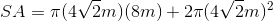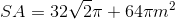# High School Math : How to find the surface area of a polyhedron

## Example Questions

### Example Question #1 : Other Polyhedrons

Find the surface area of the following half-cylinder.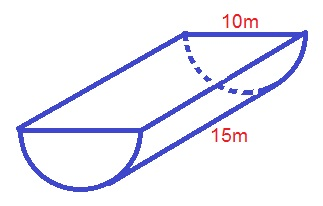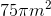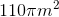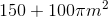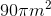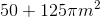Explanation:

The formula for the surface area of a half-cylinder must include one-half of the surface area of a cylinder, which would be: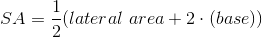We also need to add the area of the new rectangular face that is created by cutting the cylinder in half. The area of this rectangle would be: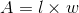where the length of the rectangle is the same as the height of the half-cylinder, and the width of the rectangle is the same as the diameter of the base of the half-cylinder. So we can rewrite the area of the rectangle as: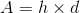Now we can combine the two area formulas to find the total surface area of the half-cylinder: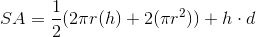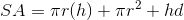whereis the radius of the base andis the length of the height, andis the diameter of the base.

Plugging in our values, we get: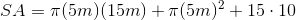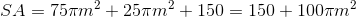### Example Question #21 : Solid Geometry

Find the surface area of the following polyhedron.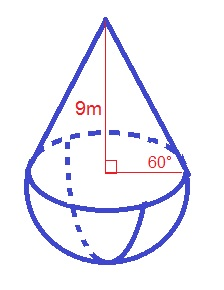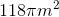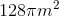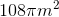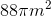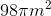Explanation:

The formula for the surface area of the polyhedron is: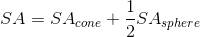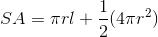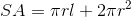Whereis the radius of the cone,is the slant height of the cone, andis the radius of the sphere

Use the formula for a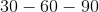triangle to find the radius and slant height: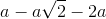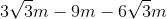Plugging in our values, we get: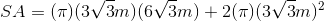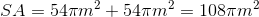### Example Question #22 : Solid Geometry

Find the surface area of the following polyhedron.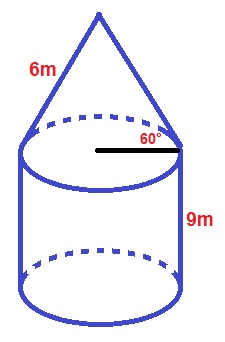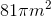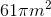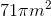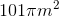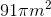Explanation:

The formula for the surface area of the polyhedron is: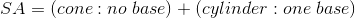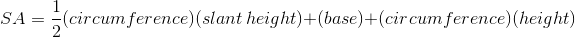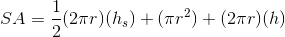whereis the radius of the cone,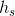is the slant height of the cone,is the radius of the cylinder, andis the height of the cylinder.

Use the formula for atriangle to find the length of the radius: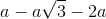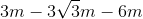Plugging in our values, we get: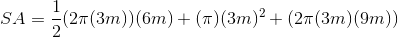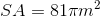### Example Question #23 : Solid Geometry

Find the surface area of the following polyhedron.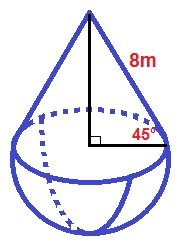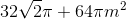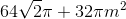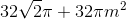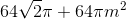Explanation:

The formula for the surface area of a polyhedron is: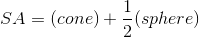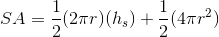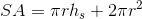whereis the radius of the polyhedron andis the slant height of the cone.

Use the formula for a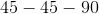triangle to find the length of the radius: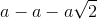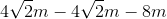Plugging in our values, we get: